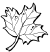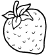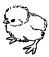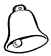Create a new printableMath Worksheets
Division

Sample - Click above to make a new math worksheet (PDF).
 Name _____________________________Date ___________________
Division
Complete.

1.
Divide 12 leaves into groups of 3.____ groups _____ left over 12  ÷  3  =  ____
2.
Divide 12 strawberries into groups of 2.____ groups _____ left over 12  ÷  2  =  ____
3.
Divide 12 birds into groups of 4.____ groups _____ left over 12  ÷  4  =  ____
 4 * This is a pre-made sheet.Use the link at the top of the page for a printable page.
5.
Divide 11 bells into groups of 4.____ groups _____ left over 8  ÷  4  =  ____